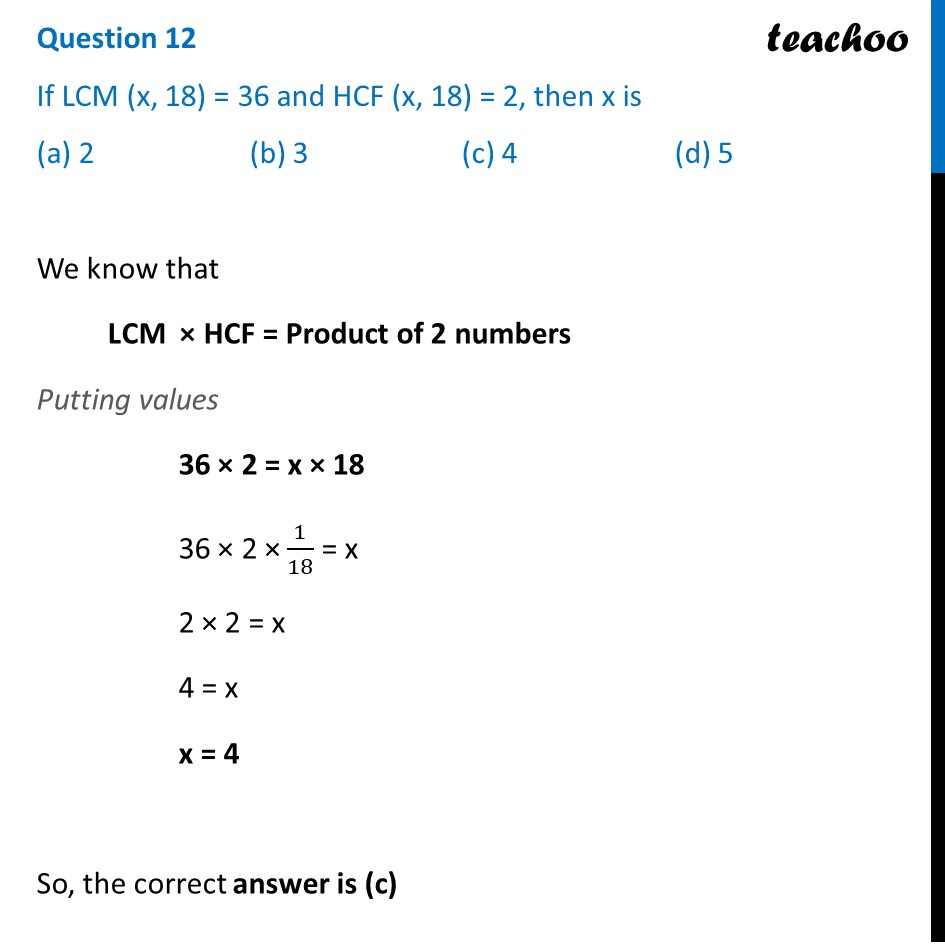CBSE Class 10 Sample Paper for 2022 Boards - Maths Standard [MCQ]

Class 10
Solutions of Sample Papers for Class 10 Boards

## (a) 2   (b) 3   (c) 4   (d) 5

This question is inspired from Question 1 (Choice - 1) - CBSE Class 10 Sample Paper for 2021 Boards - Maths StandardLearn in your speed, with individual attention - Teachoo Maths 1-on-1 Class

### Transcript

Question 12 If LCM (x, 18) = 36 and HCF (x, 18) = 2, then x is (a) 2 (b) 3 (c) 4 (d) 5 We know that LCM × HCF = Product of 2 numbers Putting values 36 × 2 = x × 18 36 × 2 × 1/18 = x 2 × 2 = x 4 = x x = 4 So, the correct answer is (c)